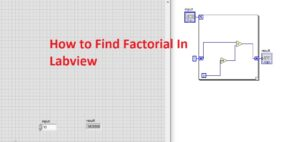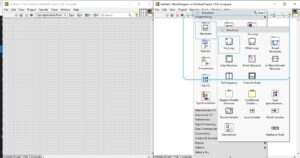Hello, readers welcome to a new post in this post we will have a detailed look at How to Find Factorial In Labview. In this LabVIEW simulation, we will create a factorial of numbers by providing a certain number at the input and in results will find its factorial. Here is factorial of any number is that 5! its factorial will be 1*2*3*4*5=120. This form we will find in the Labview software practically.

So let’s get started.

## How to Find Factorial In Labview

• First of all, open the LabVIEW software on your computer. Click right of your mouse in the control pallet and choose a numeric option and then lick at controls.

• Then move to block diagram click right and from the function palette choose the structure option and then click at for loop. We will use for loop to make our factorial program in the Labview.• Make interlinking between numeric control where we will save input given to the N of loop that explains how many time our function will run.• In this program, i am going to use for loop but there is an option for while loop usage.
• In a factorial, all numbers are multiplied to get factorial for this purpose we will use shift registers to use this option click right at the for loop wall and there will be a window open from that select shift register.• There is a need of operates or initialize the shift register. To do this choose the numeric from function pallet and after that choose constant can see here.
• Link this numeric to the shift register and adjust the value of one.

• Here we put one as starting number in place of zero if you place there will be zero results as in factorial all numbers will be multiplied so choose one.
• For the multiplication process put multiply tab through the function palette and choose numeric and put multiply tab.• At the right shift register press right and choose to create and then select indicator can see here.
• Here you can see the net circuit of factorial operation to find factorial can seen here.

Just place the values of numbers for which you want to find factorial and in results, you will get factorial of that number.So friends that is a detailed post about How to Find Factorial In Labview if you have any further query ask in the comments. Thanks for reading have a nice day.